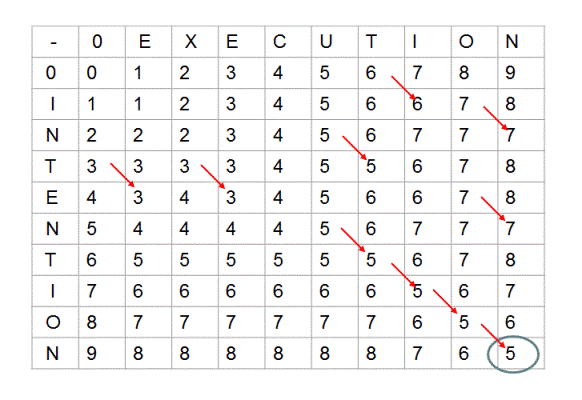[算法笔记 2] 动态规划• 乌龟棋
• $pos \leftarrow i+j \times 2+k \times 3+l \times 4$
• $dp [i+1][j][k][l]=dp [i][j][k][l]+s [pos+1]$
• $dp [i][j+1][k][l]=dp [i][j][k][l]+s [pos+2]…$ 以此类推
• Codeforces 1114D. Flood Fill
• $dp [l-1][r]=\min \{ dp [l][r] + diff (a [l - 1], a [l]), dp [l][r] + diff (a [l - 1], a [r]) \}$
• $dp [l][r+1]=\min \{ dp [l][r] + diff (a [r + 1], a [r]), dp [l][r] + diff (a [r + 1], a [l]) \}$
• Codeforces 1132F. Clear the String
• $dp [l][r] = \max \{1 + dp [l + 1][r], \min \limits_{l < k \le r, s_k = s_r} dp [l + 1][k - 1] + dp [k][r] \}$